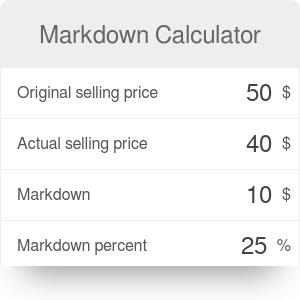Cost
\$
Markup
%
Margin
%
Minimal revenue
\$
Minimal profit
\$
Markdown
%
Markdown amount
\$
List price
\$

# Markdown Calculator

By Bogna Haponiuk

If you work in sales, you will probably appreciate our markdown calculator. It will help you to determine the list price that, after a given markdown, will result in an expected markup or profit margin. If you are not sure what do these terms mean, just keep reading to discover their definitions and a step-by-step tutorial of how to calculate the markdown price.

## What is markdown?

Markdown is the lowering of the product price, basing on the inability to sell it at the initial price. For example, a shirt in a clothing shop can initially cost \$50. If almost no one wants to buy it at this price, it is common that a markdown (for example of 20%) is introduced. After the markdown, the shirt costs only \$40 and while the shop owner will have a lower profit on each sold shirt, he might actually sell more shirts that before.

Markdown is a bit different from a discount, which is usually offered to a specific group of people such as students or frequent buyers. While markdowns are offered to everyone, discounts make customers feel they are treated in a special way.

## Markdown calculation process

Our markdown calculator finds all data necessary in the sales process for you. All you have to do is to input any three of the values listed below to have a full overview of the process.

• Cost: the production cost of one product. For example, the production of one shirt in your company might cost \$10.
• Markup: a ratio of profit to cost expressed as a percentage. For example, you can decide that you want a markup of 100% on each of your products - that means that the profit you will make on each item is equal to its production cost.
• Margin: the ratio of profit to revenue, also expressed as a percentage. What is the difference between markup and margin? Revenue is the price of your product, so it is the sum of production cost and profit. The margin will always be lower than the markup.
• Minimal revenue: the price of your product - how much money flows into your pocket per each item, not taking production costs into account.
• Minimal profit: the net profit you make on each of your products. It is the revenue after subtracting production costs from it.
• Markdown: as stated before, this is the percentage lowering of the price of your product.
• Markdown amount: the amount of money your customers will save on the product.
• List price: initial price of the item. After you deduct the markdown amount from this price you will get your revenue.

## How to calculate markdown price: an example

Let's assume you produce clothes. The cost of producing one shirt is, as assumed earlier, \$10. You want to give your customers a 30% markdown, at the same time keeping a 50% markup on the item.

1. Begin with calculating your expected profit. It will be equal to markup multiplied by production cost, so `100% * \$10 = \$10`.
2. Then, calculate the revenue as the sum of production cost and profit: `\$20 + \$10 = \$20`.
3. You can also find the gross margin as the proportion between profit and revenue: `\$10 / \$20 = 50%`.
4. Once you know these values, you can calculate the initial (list) price of the shirt. You know that after the markdown the price of the shirt will be equal to your revenue. Hence, the list price is equal to revenue divided by (100% - markdown): `\$20 / (100% - 30%) = \$20 / 0.7 = \$28.57`.
5. Now, you can find the last value - the markdown amount - as the difference between the list price and revenue: `\$28.57 - \$20 = \$8.57`.

You have just calculated that you have to give the list price of the item as \$28.57. After some time, you can give your customers a markdown of 30% and still have a 100% markup on the product.

For more complicated sales cases, you can always check our markup and margin calculator for 2 sets.

Bogna Haponiuk

## Get the widget!

Markdown Calculator can be embedded on your website to enrich the content you wrote and make it easier for your visitors to understand your message.

It is free, awesome and will keep people coming back!